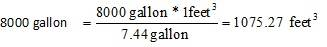# A cubic foot is equal 7.48 gallons. How many cubic feet wil

• jim1174
Just be more careful.ok i think i got itIn summary, to find the number of cubic feet a tank can hold based on its volume in gallons, you first need to know the conversion factor of 1 cubic foot to gallons, which is 7.48 gallons. Then, using the given volume in gallons, you can calculate the number of cubic feet by dividing the volume in gallons by the conversion factor of 7.48 gallons per cubic foot. In this case, the tank with a volume of 8000 gallons will hold approximately 1075.27 cubic feet. It is important to be careful with units and conversion factors to avoid making mistakes.

## Homework Statement

2. A cubic foot is equal 7.48 gallons. How many cubic feet will the tank describe in question #6 hold?

## The Attempt at a Solution

Tank has a volume of 8000 gallons.

1 feet3 = 7.44 gallons (given)

Therefore,

8000 gallon = 8000gallon x feet3/7.44gallon= 107527feet

jim1174 said:

## Homework Statement

2. A cubic foot is equal 7.48 gallons. How many cubic feet will the tank describe in question #6 hold?

## The Attempt at a Solution

Tank has a volume of 8000 gallons.

1 feet3 = 7.44 gallons (given)

Therefore,

8000 gallon = 8000gallon x feet3/7.44gallon= 107527feet
Your answer should be in units of cubic feet, not feet (usually written as cu. ft of ft3). When you write exponents, at the very least use ^ to indicate an exponent.

Your numerical answer is way off. Without getting bogged down too much with numbers, let's say for the purpose of a rough calculation that a gallon is equal to 8 cu. ft. Without using a calculator, how many cubic feet would this be? You should be able to do this in your head.

Mark44 said:
Your answer should be in units of cubic feet, not feet (usually written as cu. ft of ft3). When you write exponents, at the very least use ^ to indicate an exponent.

Your numerical answer is way off. Without getting bogged down too much with numbers, let's say for the purpose of a rough calculation that a gallon is equal to 8 cu. ft. Without using a calculator, how many cubic feet would this be? You should be able to do this in your head.
Actually, a gallon is ~1/8 cu. ft.

But, jim1174, do you really think that 8000/7.48 > 100000 ?

Chet

I must have written that without thinking very hard. Yes, a cubic foot is about 8 gallons, so 1 gal. ##\approx## 1/8 cu. ft.

ok i think i got itjim1174 said:
ok i think i got itIt depends. In your first post you have 7.44 gal./cu. ft in one place and 7.48 gal./cu. ft. in another place. Which is it?

You need to be more careful. I've seen several mistakes like this in your work.